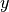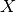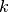Shortcuts

# Classic ML Models¶

This module implements classic machine learning models in PyTorch Lightning, including linear regression and logistic regression. Unlike other libraries that implement these models, here we use PyTorch to enable multi-GPU, multi-TPU and half-precision training.

## Linear Regression¶

Linear regression fits a linear model between a real-valued target variableand one or more features. We estimate the regression coefficients that minimize the mean squared error between the predicted and true target values.

We formulate the linear regression model as a single-layer neural network. By default we include only one neuron in the output layer, although you can specify the output_dim yourself.

Add either L1 or L2 regularization, or both, by specifying the regularization strength (default 0).

from pl_bolts.models.regression import LinearRegression
import pytorch_lightning as pl
from pl_bolts.datamodules import SklearnDataModule

model = LinearRegression(input_dim=13)
trainer = pl.Trainer()

class pl_bolts.models.regression.linear_regression.LinearRegression(input_dim, output_dim=1, bias=True, learning_rate=0.0001, optimizer=torch.optim.Adam, l1_strength=0.0, l2_strength=0.0, **kwargs)[source]

Bases: pytorch_lightning.

Linear regression model implementing - with optional L1/L2 regularization $$min_{W} ||(Wx + b) - y ||_2^2$$

Parameters

## Logistic Regression¶

Logistic regression is a linear model used for classification, i.e. when we have a categorical target variable. This implementation supports both binary and multi-class classification.

In the binary case, we formulate the logistic regression model as a one-layer neural network with one neuron in the output layer and a sigmoid activation function. In the multi-class case, we use a single-layer neural network but now withneurons in the output, whereis the number of classes. This is also referred to as multinomial logistic regression.

Add either L1 or L2 regularization, or both, by specifying the regularization strength (default 0).

from sklearn.datasets import load_iris
from pl_bolts.models.regression import LogisticRegression
from pl_bolts.datamodules import SklearnDataModule
import pytorch_lightning as pl

# use any numpy or sklearn dataset
dm = SklearnDataModule(X, y)

# build model
model = LogisticRegression(input_dim=4, num_classes=3)

# fit
trainer = pl.Trainer(tpu_cores=8, precision=16)



Any input will be flattened across all dimensions except the first one (batch). This means images, sound, etc… work out of the box.

# create dataset
dm = MNISTDataModule(num_workers=0, data_dir=tmpdir)

model = LogisticRegression(input_dim=28 * 28, num_classes=10, learning_rate=0.001)
model.prepare_data = dm.prepare_data

trainer = pl.Trainer(max_epochs=2)
trainer.fit(model)
trainer.test(model)
# {test_acc: 0.92}

class pl_bolts.models.regression.logistic_regression.LogisticRegression(input_dim, num_classes, bias=True, learning_rate=0.0001, optimizer=torch.optim.Adam, l1_strength=0.0, l2_strength=0.0, **kwargs)[source]

Bases: pytorch_lightning.

Logistic regression model.

Parameters

© Copyright Copyright (c) 2020-2021, PyTorchLightning et al.. Revision 2f93c882.

Built with Sphinx using a theme provided by Read the Docs.
Versions
latest
stable
0.4.0
0.3.4
0.3.3
0.3.2
0.3.1
0.3.0
0.2.5
0.2.4
0.2.3
0.2.2
0.2.1
0.2.0
0.1.1
docs-build-rtd
0.1.0